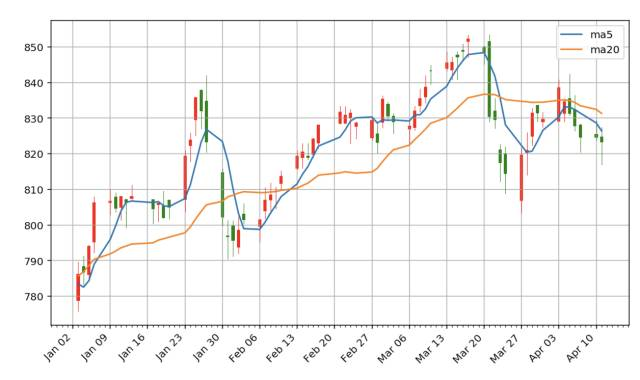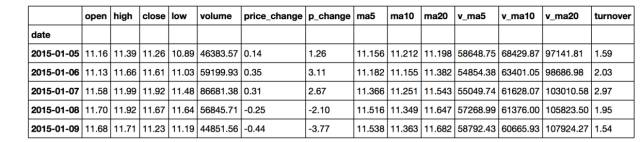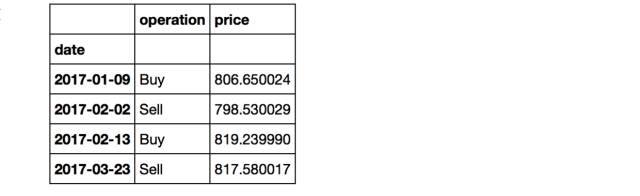# 用 Python 浅析股票数据import pandas as pd

import numpy as np

import matplotlib.pyplot as plt

%matplotlib inline

%config InlineBackend.figure_format = 'retina'

%pylab inline

pylab.rcParams['figure.figsize'] = (10, 6) #设置绘图尺寸

#读取数据

stock = pd.read_table('stockData.txt', usecols=range(15), parse_dates=, index_col=0)

stock = stock[::-1]  #逆序排列stock.info()

<class 'pandas.core.frame.DataFrame'>

DatetimeIndex: 20 entries, 2015-01-05 to 2015-01-30

Data columns (total 14 columns):

open        20 non-null float64

high            20 non-null float64

close           20 non-null float64

low             20 non-null float64

volume          20 non-null float64

price_change    20 non-null float64

p_change        20 non-null float64

ma5             20 non-null float64

ma10            20 non-null float64

ma20            20 non-null float64

v_ma5           20 non-null float64

v_ma10          20 non-null float64

v_ma20          20 non-null float64

turnover        20 non-null float64

dtypes: float64(14)

memory usage: 2.3 KB

stock.columns

Index(['    open', 'high', 'close', 'low', 'volume', 'price_change',

'p_change', 'ma5', 'ma10', 'ma20', 'v_ma5', 'v_ma10', 'v_ma20',

'turnover'],

dtype='object')

stock.rename(columns={'    open':'open'}, inplace=True)• 价格相关指标
• 当日价格：开盘、收盘价，最高、最低价
• 价格变化：价格变动和涨跌幅
• 均价：5、10、20日均价
• 成交量相关指标
• 成交量
• 换手率：成交量/发行总股数×100%
• 成交量均量：5、10、20日均量

stock['close'].plot(grid=True)K线图Matplotlib.finance模块提供了绘制K线图的函数candlestick_ohlc()，但如果要绘制比较美观的K线图还是要下点功夫的。下面定义了pandas_candlestick_ohlc()函数来绘制适用于本文数据的K线图，其中大部分代码都是在设置坐标轴的格式。

from matplotlib.finance import candlestick_ohlc

from matplotlib.dates import DateFormatter, WeekdayLocator, DayLocator, MONDAY

def pandas_candlestick_ohlc(stock_data, otherseries=None):

# 设置绘图参数，主要是坐标轴

mondays = WeekdayLocator(MONDAY)

alldays = DayLocator()

dayFormatter = DateFormatter('%d')

fig, ax = plt.subplots()

if stock_data.index[-1] - stock_data.index < pd.Timedelta('730 days'):

weekFormatter = DateFormatter('%b %d')

ax.xaxis.set_major_locator(mondays)

ax.xaxis.set_minor_locator(alldays)

else:

weekFormatter = DateFormatter('%b %d, %Y')

ax.xaxis.set_major_formatter(weekFormatter)

ax.grid(True)

# 创建K线图

stock_array = np.array(stock_data.reset_index()[['date','open','high','low','close']])

stock_array[:,0] = date2num(stock_array[:,0])

candlestick_ohlc(ax, stock_array, colorup = "red", colordown="green", width=0.4)

# 可同时绘制其他折线图

if otherseries is not None:

for each in otherseries:

plt.plot(stock_data[each], label=each)

plt.legend()

ax.xaxis_date()

ax.autoscale_view()

plt.setp(plt.gca().get_xticklabels(), rotation=45, horizontalalignment='right')

plt.show()

pandas_candlestick_ohlc(stock)stock['return'] = stock['close'] / stock.close.iloc

stock['return'].plot(grid=True)stock['p_change'].plot(grid=True).axhline(y=0, color='black', lw=2)close_price = stock['close']

log_change = np.log(close_price) - np.log(close_price.shift(1))

log_change.plot(grid=True).axhline(y=0, color='black', lw=2)small = stock[['close', 'price_change', 'ma20','volume', 'v_ma20', 'turnover']]

_ = pd.scatter_matrix(small)small = stock[['close', 'price_change', 'ma20','volume', 'v_ma20']]

cov = np.corrcoef(small.T)

cov

array([[ 1.        ,  0.30308764,  0.10785519,  0.91078009, -0.37602193],

[ 0.30308764,  1.        , -0.45849273,  0.3721832 , -0.25950305],

[ 0.10785519, -0.45849273,  1.        , -0.06002202,  0.51793654],

[ 0.91078009,  0.3721832 , -0.06002202,  1.        , -0.37617624],

[-0.37602193, -0.25950305,  0.51793654, -0.37617624,  1.        ]])

img = plt.matshow(cov,cmap=plt.cm.winter)

plt.colorbar(img, ticks=[-1,0,1])

plt.show()stock[['close','volume']].plot(secondary_y='volume', grid=True)import datetime

# 设置股票数据的时间跨度

start = datetime.datetime(2016,10,1)

end = datetime.date.today()

goog = web.DataReader("GOOG", "yahoo", start, end)

#修改索引和列的名称，以适应本文的分析

goog.index.rename('date', inplace=True)

goog.rename(columns={'Open':'open', 'High':'high', 'Low':'low', 'Close':'close'}, inplace=True)goog["ma5"] = np.round(goog["close"].rolling(window = 5, center = False).mean(), 2)

goog["ma20"] = np.round(goog["close"].rolling(window = 20, center = False).mean(), 2)

goog = goog['2017-01-01':]

pandas_candlestick_ohlc(goog, ['ma5','ma20'])goog['ma5-20'] = goog['ma5'] - goog['ma20']

goog['diff'] = np.sign(goog['ma5-20'])

goog['diff'].plot(ylim=(-2,2)).axhline(y=0, color='black', lw=2)goog['signal'] = np.sign(goog['diff'] - goog['diff'].shift(1))

goog['signal'].plot(ylim=(-2,2))pd.DataFrame({"price": goog.loc[goog["signal"] == 1, "close"],

pd.DataFrame({"price": goog.loc[goog["signal"] == -1, "close"],

"operation": "Sell"})

])• An Introduction to Stock Market Data Analysis with Python (Part 1)
• An Introduction to Stock Market Data Analysis with Python (Part 2)
• K线理论
• K线图做图示例

##### 历史上的今天
5月
21• 版权声明 本文源自 鱼心DrFish 整理 发表于 2017年5月21日17:12:41